# bodemag

Magnitude-only Bode plot of frequency response

## Syntax

``bodemag(sys)``
``bodemag(sys1,sys2,...,sysN)``
``bodemag(sys1,LineSpec1,...,sysN,LineSpecN)``
``bodemag(___,w)``

## Description

`bodemag` enables you to generate magnitude-only plots to visualize the magnitude frequency response of a dynamic system.

For a more comprehensive function, see `bode`. `bode` provides magnitude and phase information. If you have System Identification™ toolbox, `bode` also returns the computed values, including statistical estimates.

For more customizable plotting options, see `bodeplot`.

example

````bodemag(sys)` creates a Bode magnitude plot of the frequency response of the dynamic system model `sys`. The plot displays the magnitude (in dB) of the system response as a function of frequency. `bodemag` automatically determines frequencies to plot based on system dynamics. If `sys` is a multi-input, multi-output (MIMO) model, then `bodemag` produces an array of Bode magnitude plots in which each plot shows the frequency response of one I/O pair. ```

example

````bodemag(sys1,sys2,...,sysN)` plots the frequency response of multiple dynamic systems on the same plot. All systems must have the same number of inputs and outputs.```

example

````bodemag(sys1,LineSpec1,...,sysN,LineSpecN)` specifies a color, line style, and marker for each system in the plot.```

example

````bodemag(___,w)` plots system responses for frequencies specified by `w`.If `w` is a cell array of the form `{wmin,wmax}`, then `bodemag` plots the response at frequencies ranging between `wmin` and `wmax`.If `w` is a vector of frequencies, then `bodemag` plots the response at each specified frequency.You can use this syntax with any of the input-argument combinations in previous syntaxes.```

## Examples

collapse all

Create a Bode magnitude plot of the following continuous-time SISO dynamic system.

`$\mathit{H}\left(\mathit{s}\right)=\frac{{\mathit{s}}_{}^{2}+0.1\mathit{s}+7.5}{{\mathit{s}}_{}^{4}+0.12{\mathit{s}}_{}^{3}+9{\mathit{s}}_{}^{2}}$`

```H = tf([1 0.1 7.5],[1 0.12 9 0 0]); bodemag(H)```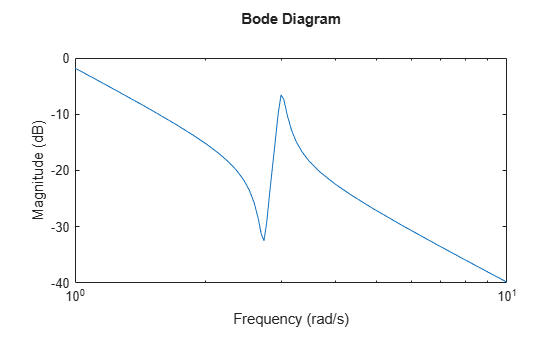`bodemag` automatically selects the plot range based on the system dynamics.

Create a Bode magnitude plot over a specified frequency range. Use this approach when you want to focus on the dynamics in a particular range of frequencies.

```H = tf([-0.1,-2.4,-181,-1950],[1,3.3,990,2600]); bodemag(H,{1,100}) grid on```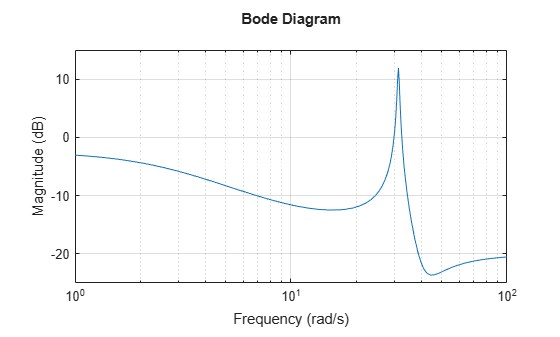The cell array `{1,100}` specifies the minimum and maximum frequency values in the Bode magnitude plot. When you provide frequency bounds in this way, the function selects intermediate points for frequency response data.

Alternatively, specify a vector of frequency points to use for evaluating and plotting the frequency response.

```w = [1 5 10 15 20 23 31 40 44 50 85 100]; bodemag(H,w,'.-') grid on```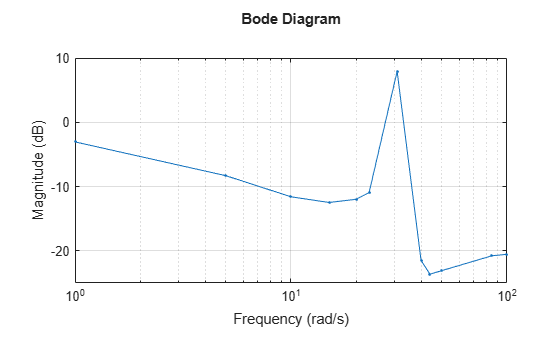`bodemag` plots the frequency response at the specified frequencies only.

Compare the magnitude of the frequency response of a continuous-time system to an equivalent discretized system on the same Bode plot.

Create continuous-time and discrete-time dynamic systems.

```H = tf([1 0.1 7.5],[1 0.12 9 0 0]); Hd = c2d(H,0.5,'zoh');```

Create a Bode magnitude plot that displays the responses of both systems.

`bodemag(H,Hd)`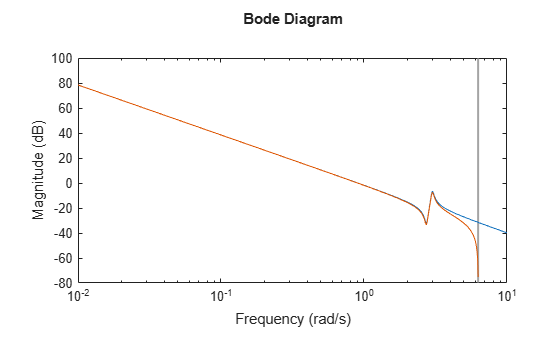The Bode magnitude plot of a discrete-time system includes a vertical line marking the Nyquist frequency of the system.

Specify the color, linestyle, or marker for each system in a Bode magnitude plot using the `LineSpec` input arguments.

```H = tf([1 0.1 7.5],[1 0.12 9 0 0]); Hd = c2d(H,0.5,'zoh'); bodemag(H,'r',Hd,'b--')```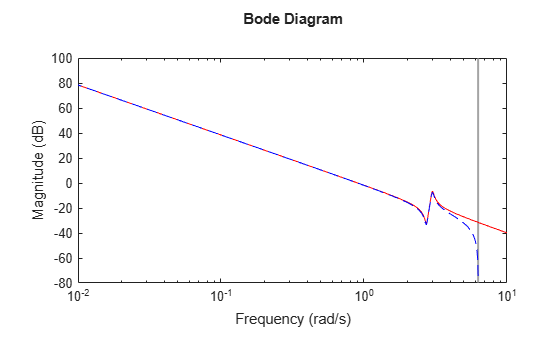The first `LineSpec` argument `'r'` specifies a solid red line for the response of `H`. The second `LineSpec` argument `'b--'` specifies a dashed blue line for the response of `Hd`.

For this example, create a 2-output, 3-input system.

```rng(0,'twister'); % For reproducibility H = rss(4,2,3);```

For this system, `bodemag` plots the magnitude-only frequency responses of each I/O channel in a separate plot in a single figure.

`bodemag(H)`## Input Arguments

collapse all

Dynamic system, specified as a SISO or MIMO dynamic system model or array of dynamic system models. Dynamic systems that you can use include:

• Continuous-time or discrete-time numeric LTI models, such as `tf` (Control System Toolbox), `zpk` (Control System Toolbox), or `ss` (Control System Toolbox) models.

• Generalized or uncertain LTI models such as `genss` (Control System Toolbox) or `uss` (Robust Control Toolbox) models. (Using uncertain models requires Robust Control Toolbox™ software.)

• For tunable control design blocks, the function evaluates the model at its current value for both plotting and returning frequency response data.

• For uncertain control design blocks, the function plots the nominal value and random samples of the model. When you use output arguments, the function returns frequency response data for the nominal model only.

• Frequency-response data models such as `frd` models. For such models, the function plots the response at frequencies defined in the model.

• Identified LTI models, such as `idtf`, `idss`, or `idproc` models.

If `sys` is an array of models, the function plots the frequency responses of all models in the array on the same axes.

Line style, marker, and color, specified as a string or vector of one, two, or three characters. The characters can appear in any order. You do not need to specify all three characteristics (line style, marker, and color). For example, if you omit the line style and specify the marker, then the plot shows only the marker and no line. For more information about configuring this argument, see the `LineSpec` input argument of the `plot` function.

Example: `'r--'` specifies a red dashed line

Example: `'*b'` specifies blue asterisk markers

Example: `'y'` specifies a yellow line

Frequencies at which to compute and plot frequency response, specified as the cell array `{wmin,wmax}` or as a vector of frequency values.

• If `w` is a cell array of the form `{wmin,wmax}`, then the function computes the index at frequencies ranging between `wmin` and `wmax`.

• If `w` is a vector of frequencies, then the function computes the index at each specified frequency. For example, use `logspace` to generate a row vector with logarithmically spaced frequency values.

Specify frequencies in units of rad/`TimeUnit`, where `TimeUnit` is the `TimeUnit` property of the model.

## Algorithms

`bodemag` computes the frequency response as follows:

1. Compute the zero-pole-gain (`zpk` (Control System Toolbox)) representation of the dynamic system.

2. Evaluate the gain and phase of the frequency response based on the zero, pole, and gain data for each input/output channel of the system.

• For continuous-time systems, `bodemag` evaluates the frequency response on the imaginary axis s = and considers only positive frequencies.

• For discrete-time systems, `bodemag` evaluates the frequency response on the unit circle. To facilitate interpretation, the command parameterizes the upper half of the unit circle as:

`$z={e}^{j\omega {T}_{s}},\text{ }0\le \omega \le {\omega }_{N}=\frac{\pi }{{T}_{s}},$`

where Ts is the sample time and ωN is the Nyquist frequency. The equivalent continuous-time frequency ω is then used as the x-axis variable. Because $H\left({e}^{j\omega {T}_{s}}\right)$ is periodic with period 2ωN, `bodemag` plots the response only up to the Nyquist frequency ωN. If `sys` is a discrete-time model with unspecified sample time, `bodemag` uses Ts = 1.

## Version History

Introduced in R2012a